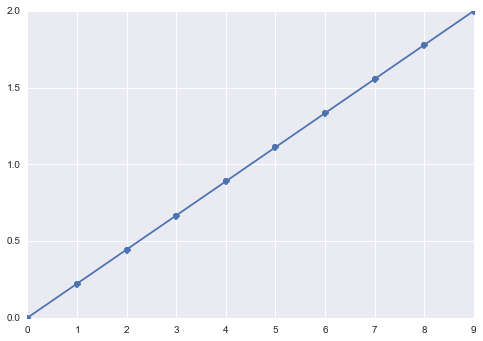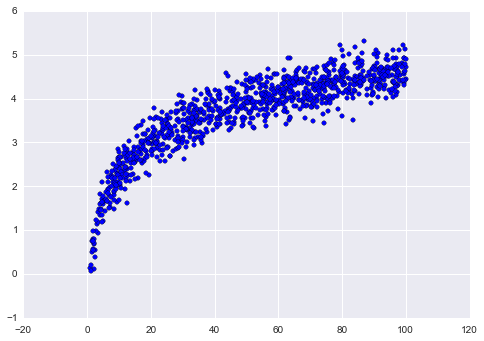# NumPy¶

Credits: Forked from Parallel Machine Learning with scikit-learn and IPython by Olivier Grisel

• NumPy Arrays, dtype, and shape
• Common Array Operations
• Reshape and Update In-Place
• Combine Arrays
• Create Sample Data
In :
import numpy as np


## NumPy Arrays, dtypes, and shapes¶

In :
a = np.array([1, 2, 3])
print(a)
print(a.shape)
print(a.dtype)

[1 2 3]
(3,)
int64

In :
b = np.array([[0, 2, 4], [1, 3, 5]])
print(b)
print(b.shape)
print(b.dtype)

[[0 2 4]
[1 3 5]]
(2, 3)
int64

In :
np.zeros(5)

Out:
array([ 0.,  0.,  0.,  0.,  0.])
In :
np.ones(shape=(3, 4), dtype=np.int32)

Out:
array([[1, 1, 1, 1],
[1, 1, 1, 1],
[1, 1, 1, 1]], dtype=int32)

## Common Array Operations¶

In :
c = b * 0.5
print(c)
print(c.shape)
print(c.dtype)

[[ 0.   1.   2. ]
[ 0.5  1.5  2.5]]
(2, 3)
float64

In :
d = a + c
print(d)

[[ 1.   3.   5. ]
[ 1.5  3.5  5.5]]

In :
d

Out:
array([ 1.,  3.,  5.])
In :
d[0, 0]

Out:
1.0
In :
d[:, 0]

Out:
array([ 1. ,  1.5])
In :
d.sum()

Out:
19.5
In :
d.mean()

Out:
3.25
In :
d.sum(axis=0)

Out:
array([  2.5,   6.5,  10.5])
In :
d.mean(axis=1)

Out:
array([ 3. ,  3.5])

## Reshape and Update In-Place¶

In :
e = np.arange(12)
print(e)

[ 0  1  2  3  4  5  6  7  8  9 10 11]

In :
# f is a view of contents of e
f = e.reshape(3, 4)
print(f)

[[ 0  1  2  3]
[ 4  5  6  7]
[ 8  9 10 11]]

In :
# Set values of e from index 5 onwards to 0
e[5:] = 0
print(e)

[0 1 2 3 4 0 0 0 0 0 0 0]

In :
# f is also updated
f

Out:
array([[0, 1, 2, 3],
[4, 0, 0, 0],
[0, 0, 0, 0]])
In :
# OWNDATA shows f does not own its data
f.flags

Out:
  C_CONTIGUOUS : True
F_CONTIGUOUS : False
OWNDATA : False
WRITEABLE : True
ALIGNED : True
UPDATEIFCOPY : False

## Combine Arrays¶

In :
a

Out:
array([1, 2, 3])
In :
b

Out:
array([[0, 2, 4],
[1, 3, 5]])
In :
d

Out:
array([[ 1. ,  3. ,  5. ],
[ 1.5,  3.5,  5.5]])
In :
np.concatenate([a, a, a])

Out:
array([1, 2, 3, 1, 2, 3, 1, 2, 3])
In :
# Use broadcasting when needed to do this automatically
np.vstack([a, b, d])

Out:
array([[ 1. ,  2. ,  3. ],
[ 0. ,  2. ,  4. ],
[ 1. ,  3. ,  5. ],
[ 1. ,  3. ,  5. ],
[ 1.5,  3.5,  5.5]])
In :
# In machine learning, useful to enrich or
# add new/concatenate features with hstack
np.hstack([b, d])

Out:
array([[ 0. ,  2. ,  4. ,  1. ,  3. ,  5. ],
[ 1. ,  3. ,  5. ,  1.5,  3.5,  5.5]])

## Create Sample Data¶

In :
%matplotlib inline

import pylab as plt
import seaborn

seaborn.set()

In :
# Create evenly spaced numbers over the specified interval
x = np.linspace(0, 2, 10)
plt.plot(x, 'o-');
plt.show()In :
# Create sample data, add some noise
x = np.random.uniform(1, 100, 1000)
y = np.log(x) + np.random.normal(0, .3, 1000)

plt.scatter(x, y)
plt.show()